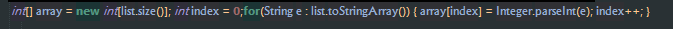# How can i ask list in extension

which is my first extension two months ago from

https://medium.com/@saitwalshreyash19/writing-your-first-app-inventor-2-extension-dc6d5d4ff824

by @shreyash now once i learnt some what on #open-source-development #extensions
now i want to input many colors

actual code

@SimpleFunction(description = "this block is where u put ur component,colors,u may have dout what is orientation it is where u put the green block i gave in gradients section")
public void SetGradientBackground3color(AndroidViewComponent component, Object orientation, int color1, int color2) {

``````// To set gradient colors as background, what we are actually going to
// do is create a new GradientDrawable object and set it as
// the background of the view. With this approach, it'd be possible to
// create gradients programmatically without editing the manifest
// file which is currently not supported in App Inventor 2.

// Getting the reference to the View of the parameter component using
// the getView() method of AndroidNonVisible class.
View view = component.getView();

// GradientDrawable is a subclass of Drawable class from Android
// support library. It is mostly used to create gradients.
new int[] {color1, color2});

// Setting the gradientDrawable as the background of view.
``````

}

how i converted to 3 colors

in this code at this line

new int[] {color1, color2});

i just changed

``````       new int[] {color1, color2});
``````

to

new int[] {color1, color2, color3});

and

@SimpleFunction(description = "this block is where u put ur component,colors,u may have dout what is orientation it is where u put the green block i gave in gradients section")
public void SetGradientBackground3color(AndroidViewComponent component, Object orientation, int color1, int color2) {

to

@SimpleFunction(description = "this block is where u put ur component,colors,u may have dout what is orientation it is where u put the green block i gave in gradients section")
public void SetGradientBackground3color(AndroidViewComponent component, Object orientation, int color1, int color2,int color3) {

for 3 colors it works perfectly

now my goal is to achieve unlimited colors

so what i did

i first

@SimpleFunction(description = "")
public void SetGradientBackground2color(AndroidViewComponent component, Object orientation,int color1, int color2) {

converted this line to

@SimpleFunction(description = "")
public void SetGradientBackground2color(AndroidViewComponent component, Object orientation, YailList colors) {

this line as i need yail list

So what i need

but now i have a doubt how should i change my code now to put list here

``````       new int[] {color1, color2});
``````

thanks

First of all, what is the issue you are facing?

i have tried

``````       new int[] {color1, color2});
``````

to
new int[] {colors});

so my code is

@SimpleFunction(description = "this block is where u put ur component,colors,u may have dout what is orientation it is where u put the green block i gave in gradients section")
public void SetGradientBackground2color(AndroidViewComponent component, Object orientation, YailList colors) {

``````// To set gradient colors as background, what we are actually going to
// do is create a new GradientDrawable object and set it as
// the background of the view. With this approach, it'd be possible to
// create gradients programmatically without editing the manifest
// file which is currently not supported in App Inventor 2.

// Getting the reference to the View of the parameter component using
// the getView() method of AndroidNonVisible class.
View view = component.getView();

// GradientDrawable is a subclass of Drawable class from Android
// support library. It is mostly used to create gradients.
``````

new int[] {colors} );

``````// Setting the gradientDrawable as the background of view.
``````

}
and error
is
[javac] new int[] {colors} );
[javac] ^

To convert integer array:

``````@SimpleFunction
public void example(YailList list) {
int[] array = new int[list.size()];
int index = 0;
for(String e : list.toStringArray()) {
array[index] = Integer.parseInt(e);
index++;
}
}
``````

Now in the variable called `array` you have the values.

They are called arrays.

so like this
new array[] {colors} );

What are you trying to do? You have already converted into an int array.

1 Like

Just use `array` and not `new array[] {colors} );`

1 Like

got it i will try

``````GradientDrawable gradientDrawable = new GradientDrawable(
1 Like

First do as mentioned above and just use:

``````GradientDrawable gradientDrawable = new GradientDrawable(
``````
1 Like

sorry i dint see the above post i will try

Just add this code, then do:

You're trying to take list input from the user and turn it into gradient right?

yes
but its just sample code its not main extension i will use for future purpose

1 Like

my code
int[] array = new int[list.size()]; int index = 0;for(String e : list.toStringArray()) { array[index] = Integer.parseInt(e); index++; }

my error
[javac] int[] array = new int[list.size()]; int index = 0; for(String e : list.toStringArray()) { array[index] = Integer.parseInt(e); index++; }
[javac] ^
[javac] symbol: variable list
[javac] int[] array = new int[list.size()]; int index = 0; for(String e : list.toStringArray()) { array[index] = Integer.parseInt(e); index++; }
[javac] ^
[javac] symbol: variable list

How can that error occur? It should NOT occur.

1 Like

i think it is because the `;` is nearby it should be in each line end see my codeI thought it's occured for `Integer.parseInt`
my mistake1 Like

Can you please post you're new code?

i posted and once more do u need as text or image